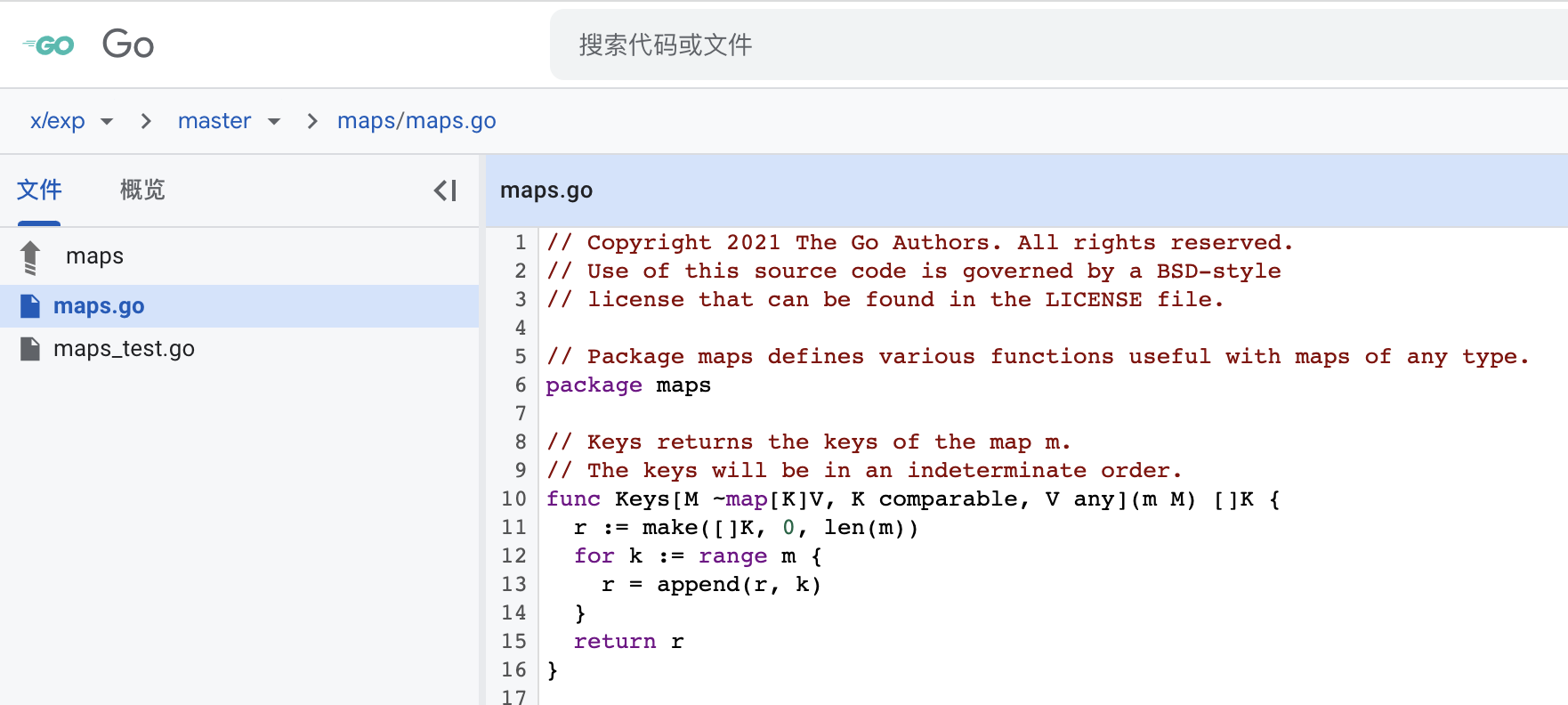# 泛型配套

## maps

Go 泛型的配套标准库 golang.org/x/exp/maps 包已经正式提交，放出来了，可以使用。``````package maps

func Keys[M ~map[K]V, K comparable, V any](m M) []K {
r := make([]K, 0, len(m))
for k := range m {
r = append(r, k)
}
return r
}

func Values[M ~map[K]V, K comparable, V any](m M) []V {
r := make([]V, 0, len(m))
for _, v := range m {
r = append(r, v)
}
return r
}

func Equal[M1, M2 ~map[K]V, K, V comparable](m1 M1, m2 M2) bool {
if len(m1) != len(m2) {
return false
}
for k, v1 := range m1 {
if v2, ok := m2[k]; !ok || v1 != v2 {
return false
}
}
return true
}

func EqualFunc[M1 ~map[K]V1, M2 ~map[K]V2, K comparable, V1, V2 any](m1 M1, m2 M2, eq func(V1, V2) bool) bool {
if len(m1) != len(m2) {
return false
}
for k, v1 := range m1 {
if v2, ok := m2[k]; !ok || !eq(v1, v2) {
return false
}
}
return true
}

func DeleteFunc[M ~map[K]V, K comparable, V any](m M, del func(K, V) bool) {
for k, v := range m {
if del(k, v) {
delete(m, k)
}
}
}
``````
• Keys：返回 map 的键值内容，键值将以不确定的顺序出现。
• Values：返回 map 的值，值将以不确定的顺序出现。
• Equal：检查两个地图是否包含相同的键/值对，内部会使用 `==` 来比较数值。
• EqualFunc：`EqualFunc``Equal` 方法类似，但使用闭包方法来比较数值，键值仍然用 `==` 来比较。
• DeleteFunc：删除 map 中闭包方法返回 `true` 的任何键/值对。
``````
func Clear[M ~map[K]V, K comparable, V any](m M) {
for k := range m {
delete(m, k)
}
}

func Clone[M ~map[K]V, K comparable, V any](m M) M {
r := make(M, len(m))
for k, v := range m {
r[k] = v
}
return r
}

func Copy[M ~map[K]V, K comparable, V any](dst, src M) {
for k, v := range src {
dst[k] = v
}
}
``````
• Clear：清除从 map 中删除所有条目，使之为空。
• Clone：返回一个 map 的副本，这是一个浅层克隆，新拷贝出来的的键和值使用普通的赋值来设置。
• Copy：复制 src 中的所有键/值对，并将其加入 dst。当 src 中的一个键已经存在于 dst 中时，dst 中的值将被与 src 中的键相关的值所覆盖。

## switch-type

### 背景

``````switch type T {
case A1:
case A2, A3:
...
}
``````

``````interface{
C
A
}
``````

``````    interface{
C
A1 | A2 | ... | An
}
``````

``````case interface {~T}:
``````

### 争议点

``````switch T.(type) {
case string:
...
default:
...
}
``````

``````switch type T {
case A1:
case A2, A3:
...
}
``````

``````type ApproxString interface { ~string }

func F[T ApproxString](v T "T ApproxString") {
switch (interface{})(v).(type) {
case string:
fmt.Println(v)
default:
panic("脑子没进煎鱼")
}
}

type MyString string

func main() {
F(MyString("脑子进煎鱼了"))
}
``````

### 案例

#### 多类型元素

``````type Stringish interface {
string | fmt.Stringer
}

func Concat[S Stringish](x []S "S Stringish") string {
switch type S {
case string:
...
case fmt.Stringer:
...
}
}
``````

#### 近似元素

``````type Constraint interface {
~int | ~int8 | ~string
}

func ThisSyntax[T Constraint]( "T Constraint") {
switch type T {
case ~int | ~int8:
...
case ~string:
...
}
}

func IsClearerThanThisSyntax[T Constraint]( "T Constraint") {
switch type T {
case interface{~int | ~int8 }:
...
case interface{ ~string }:
...
}
}
``````

## 编译减慢

### 性能分析

• -G=0 模式：默认不打开泛型的模式。
• -G=3 模式：打开泛型的模式。

Go 1.18 中的 -G=0 模式和 Go 1.17 模式的比较显示，由于非泛型的变化，编译器的速度可能降低了~1%（因为 -G=0 模式不支持泛型）。

Go 1.18 的编译时间可能比 Go 1.17 慢 15-18%，这主要是由于实现泛型所带来的变化，也就是 Go1.18 开启泛型下，编译时间会变慢。

### 差异在哪

• 在 -G=0 模式下（用于 Go 1.18 之前的所有编译器）：有一个语法分析器，创建 ir.Node 节点树的 noder 阶段，以及标准类型检查器。
• 在 -G=3 模式下：有相同的语法分析器，但程序首先由 types2（支持泛型）进行类型检查。